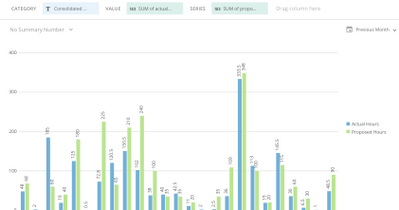# Change color when value is greater than another column

Hi,

Is there a way to change the color of a column based on it being greater than its comparison group column?  See below for the example. If the blue column is greater than the green column, I want the blue column to turn red.• HI

You need to create two beastmodes

One would be Sum of Actual hours when greater than Sum of Proposed

The other one would be Sum of Actual hours when less than Sum of Proposed

You then put the two beastmodes and Proposed hours as your fields.

Select Grouped and Stacked as Chart Type

There are a buch of toturials on this topic around

• Hi,

Can anyone help out with with request?

Thanks,

• HI

You need to create two beastmodes

One would be Sum of Actual hours when greater than Sum of Proposed

The other one would be Sum of Actual hours when less than Sum of Proposed

You then put the two beastmodes and Proposed hours as your fields.

Select Grouped and Stacked as Chart Type